International
Tables for
Crystallography
Volume F
Crystallography of biological macromolecules
Edited by M. G. Rossmann and E. Arnold

International Tables for Crystallography (2006). Vol. F, ch. 19.4, pp. 439-441   | 1 | 2 |

Section 19.4.3. Contrast variation

D. M. Engelmana* and P. B. Mooreb

aDepartment of Molecular Biophysics and Biochemistry, Yale University, New Haven, CT 06520, USA, and  bDepartments of Chemistry and Molecular Biophysics and Biochemistry, Yale University, New Haven, CT 06520, USA

19.4.3. Contrast variation

| top | pdf |

19.4.3.1. Variation of solvent density

| top | pdf |

The principle of contrast variation was studied in early work by Bragg & Perutz (1952), who observed that the magnitudes of low-order reflections in X-ray studies of protein crystals were reduced as the salt concentration in the solvent was raised. Following their concept, the effective scattering density of a dissolved particle is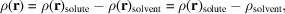whereis the average solvent scattering density. If we take a mean scattering density for the particle, a mean contrast, ρ, is defined: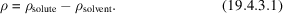If the solute and solvent have equal densities, they are `contrast matched', and the scattering from the particle vanishes at zero angle. The particle will nonetheless scatter radiation at larger angles as a consequence of internal density fluctuations, which can be useful in obtaining structural information. In the case of neutron scattering, the solvent density is most often varied by mixing D2O and H2O to obtain different percentages of D2O. This strategy gives a range of solvent densities that includes the densities of most biological molecules. However, biological molecules contain hydrogen atoms that exchange with solvent, so deuteration of the environment alters their scattering density to some extent (see below). In general, all hydrogen atoms not bonded to carbon are potentially exchangeable, but not all of these will actually exchange in a typical experiment.

To describe the variation of the radius of gyration with contrast, Stuhrmann derived the useful relationship (Stuhrmann, 1976; Stuhrmann et al., 1976)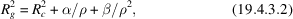which separates the contributions of the internal structure of the particle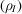to its radius of gyration from the contributions of the shape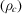. Scattering from the internal structure is independent of contrast; scattering from the shape is contrast dependent. The shape function is defined as having a value of one inside the particle and zero outside. The total scattering density is then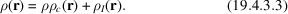The contrast-independent terms in the Stuhrmann equation are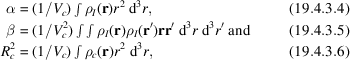where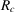is the radius of gyration of the shape function andis its volume. The sign and magnitude of α give information on the radial density distribution of scattering density in the particle: if the outer region is higher in density than the inner region, α is positive (as, for example, in lipoproteins); if the inner region is denser, α is negative. The β coefficient represents the displacement of the centre of mass as a function of the contrast and is always positive; in real cases, β is often negligible. The Stuhrmann equation leads to a useful way to represent graphically the radius of gyration data obtained from a series of contrasts: the observed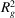is plotted versus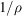. If β is negligible, the plot is a straight line of slope α, intercepting theaxis at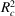. Thus,is obtained by extrapolation to a point where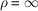, and so is often termed the radius of gyration at infinite contrast. This quantity is a representation of the shape of the particle as if it had uniform internal scattering density. In a particle with two discrete regions of density, the radius of gyration for each region can be obtained from such a graph by evaluatingwhere ρ is equal to the density of one region, so thatof the non-contrast-matched region is determined. Such measurements can also be made by adjusting the solvent to match the scattering of one region to reveal the scattering of the other.

A parameter that is often useful is the contrast-match point for the particle, which reflects its overall composition including exchange.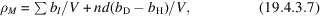where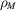, the match point, is the solvent scattering length density at which the contrast is zero and n is the number of exchanged hydrogens multiplied by d, the fractional deuteration of the water at the match point. Typically, the match point is obtained by measuring small-angle scattering at a series of D2O:H2O ratios, plotting each using a Guinier plot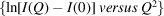to obtain a value for I(0) by extrapolation, and then plotting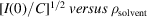, where C is the particle concentration. It is often convenient to representas per cent D2O. The plot should be a straight line, passing through zero at the contrast-match point. As noted above, the vast majority of biological molecules have contrast-match points at densities between those of H2O and D2O. If the particles are compositionally heterogeneous, the observed plot will be a weighted sum of the curves for each of the compositions present and will deviate from a straight line at low contrasts. Thus, the contrast-matching experiment can provide information on both composition and homogeneity.

While contrast variation is most often based on variation of the deuteration level in water, it is also possible to create variation by adding molecules to the solvent. As an example, a study of hydration layers was conducted by adding solute molecules, such as glycerol, at high concentration; the solute molecules alter the solvent scattering length density but do not penetrate the hydration layer (Lehmann & Zaccai, 1984).

19.4.3.2. Variation of internal contrast

| top | pdf |

A second form of contrast variation can be achieved by replacing the hydrogen atoms in biological molecules with deuterium. If, in addition, it is possible to deuterate selected regions of a particle, internal contrasts can be modified to gain information about substructures. In some cases, the opportunity arises because significant biochemical differences are present, as between the RNA and protein portions of a ribosome, the DNA and protein of a nucleosome, or the lipid and protein of a lipoprotein particle. While the intrinsic contrast may be sufficient to provide key information [as, for example, the early finding that DNA is on the outside of nucleosomes (Bradbury et al., 1976; Uberbacher et al., 1982)], it can be accentuated by incorporation of deuterated biochemical precursors or reconstitution from separately labelled components.

Internal contrast can be created in single molecules by differential incorporation of biosynthetic precursors or by chemical synthesis. Differential incorporation was first used to test models of bacteriorhodopsin, using the incorporation of deuterated amino acids supplied to a culture of halophilic archae (Engelman & Zaccai, 1980), and internal labelling was used to document conformations of cholesteryl esters that had been chemically labelled in key positions (see below). Reconstitution from purified components has been used to place deuterated proteins in ribosomes and in other complexes, again with the aim of creating internal contrast to enhance the information obtained in a neutron experiment.

In general, the creation of internal contrast can be viewed as a strategy for enriching the low information content of a solution-scattering experiment by building additional information into the sample. By design, it is known what has been labelled, so the scattering given by contrasting elements provides information about the relationships of the labelled parts to each other or to the particle as a whole. A particularly informative (but difficult) strategy is to use internal contrast to measure distances between locations in a molecule or complex. Such measurements are discussed below.

19.4.3.3. Relationship of contrasting regions

| top | pdf |

Where a particle has two regions of different scattering density, the square of the total observed radius of gyration,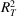, can be obtained as the weighted sum of squares of the two individual radii,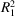and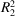, and the square of the distance between the centres of scattering mass of the two regions,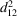, as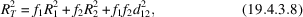where the f's are the fractions of the total scattering from each of the two regions at the solvent contrast being used in the experiment (Moore et al., 1974). By varying the contrast, a set of differently weighted equations can be obtained, from which the individual radii and the separation can be derived. This method is an alternative to the Stuhrmann analysis described above. An example of the use of this approach, based formally on the parallel axis theorem of mechanics, is found in studies of the ribosome (Moore et al., 1974). An alternative that has proved useful is to combine neutron and X-ray scattering data, since the weighting factors will differ for distinct regions, such as the RNA and protein components of the ribosome (Serdyuk et al., 1979).

19.4.3.4. The triple isotopic substitution method

| top | pdf |

An innovation in the study of subunits in a reconstituted complex was introduced by Serdyuk et al. (1994), who devised a difference method to isolate the scattering from a single subunit. The method requires three particles with different deuteration levels in the subunit: one in which the subunit is heavily deuterated (contrast with the complex = ρ), one in which the subunit is not deuterated (0), and one in which the subunit is deuterated at an intermediate level (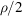):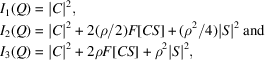where C is the scattering amplitude of the complex, S is the amplitude of the subunit, and F[CS] is the Fourier transform of the correlation function between the complex and the subunit. Scattering is measured (a) from an equimolar mixture of complexes with heavily deuterated and non-deuterated subunits and (b) from a sample of complexes with subunits with the intermediate level of deuteration. Subtraction of (b) from (a), weighted so that the two curves are equimolar, gives a net curve for the subunit alone (at half the scattering power that would be seen for a solution of the isolated subunits at the same concentration):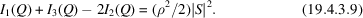The difference curve is not influenced by solvent composition, underlying order, concentration or interparticle interference effects. Thus, at the cost of some difficult biochemistry, the small-angle scattering of a subunit belonging to a large assembly can be observed in situ. In practice, the mixture is not equimolar, but is adjusted depending on the intermediate level of deuteration, relaxing some of the difficulty of the biochemistry.

19.4.3.5. Nuclear spin contrast variation

| top | pdf |

When atomic nuclei of nonzero spin are placed in a magnetic field, the spins orient. If the temperature is near absolute zero, the orientation results in a polarization that is seen by polarized neutrons, resulting in polarization-dependent scattering. Since polarized neutron sources are available, and since biological materials are rich in hydrogen, Stuhrmann has proposed and tested a measurement based on the following idea (Stuhrmann & Nierhaus, 1996). Consider a complex in which all of the hydrogen has been replaced by deuterium except in one subunit or ligand, and prepare a sample that can be frozen to T < 0.5 K, placed in a 2.5 T magnetic field and subjected to dynamic spin polarization. Scattering of polarized neutrons is measured twice, once with the hydrogen spins oriented, and once with the spins selectively depolarized using NMR saturation. The difference contains contributions from the hydrogenated region and a cross term between the region and the rest of the complex. Using a modelling approach, Stuhrmann and his colleagues have deduced a structure that locates transfer RNA molecules on a ribosome from polarized neutron data, revealing the promise of this approach (Stuhrmann & Nierhaus, 1996).

19.4.3.6. Interpretation of small-angle scattering using models

| top | pdf |

There have been many attempts to extract more information from solution-scattering experiments than the radius of gyration and forward scattering, including the distance-measuring strategies discussed below. These attempts are of two kinds: testing models and creating models. Each of these must be cast in the context of the intrinsic information content of a scattering measurement, which can be expressed in terms of the number of independent parameters, n, that can be uniquely extracted from a data set (Moore, 1980).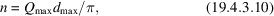where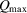is the largest Q at which statistically significant data are measured andis the largest dimension of the particle. A further requirement, normally met in small-angle scattering, is that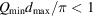.

The information content is a subtle factor in the first class of modelling, where models are tested for agreement with scattering data. Excellent programs have been written for generating predicted scattering curves from atomic coordinates and have been used to explore perturbations between crystal structures and solution organization. A fine example is the work on ATCase by Svergun, Barberato et al. (1997); the article also contains references to the programs used.

A more challenging task is to work in the other direction, extracting structural information directly from a scattering curve. Considerable effort has been devoted to work in this area, using approaches based on spherical harmonics, sometimes using sets of spheres to represent structure, and occasionally integrating information from electron microscopy (Svergun, 1994; Svergun, Burkhardt et al., 1997).

19.4.3.7. Use of forward scattering to measure molecular weights

| top | pdf |

The value of the scattering function at zero scattering angle, which is obtained by extrapolation using a Guinier plot, is related to the molecular weight of the particle. In the neutron small-angle scattering case, the incoherent scattering background from hydrogen provides an internal standard. Using the incoherent background as an absolute calibration of the beam intensity, and knowing the concentration and composition of particles, one can obtain good values for the molecular weight, as pointed out by Jacrot & Zaccai (1981) and Zaccai & Jacrot (1983). This approach applies particularly well to proteins, where the average scattering density does not vary much from case to case, and can provide important data on the stoichiometry of oligomeric complexes. The limit in the accuracy of the measurement arises from limitations in knowing the protein concentration.

References

Bradbury, E. M., Baldwin, J. P., Carpenter, B. G., Hjelm, R. P., Hancock, R. & Ibel, K. (1976). Neutron-scattering studies of chromatin. Brookhaven Symp Biol. 27, IV97–IV117.Google Scholar
Bragg, W. L. & Perutz, M. F. (1952). The external form of the haemoglobin molecule. I. Acta Cryst. 5, 277–283.Google Scholar
Engelman, D. M. & Zaccai, G. (1980). Bacteriorhodopsin is an inside-out protein. Proc. Natl Acad. Sci. USA, 77, 5894–5898.Google Scholar
Jacrot, B. & Zaccai, G. (1981). Determination of molecular weight by neutron scattering. Biopolymers, 20, 2413–2426.Google Scholar
Lehmann, M. S. & Zaccai, G. (1984). Neutron small-angle scattering studies of ribonuclease in mixed aqueous solutions and determination of the preferentially bound water. Biochemistry, 23, 1939–1942.Google Scholar
Moore, P. B. (1980). Small-angle scattering. Information content and error analysis. J. Appl. Cryst. 13, 168–175.Google Scholar
Moore, P. B., Engelman, D. M. & Schoenborn, B. P. (1974). Asymmetry in the 50S ribosomal subunit of Escherichia coli. Proc. Natl Acad. Sci. USA, 71, 172–176.Google Scholar
Serdyuk, I. N., Grenader, A. K. & Zaccai, G. (1979). Study of the internal structure of E. coli ribosomes by neutron and X-ray scattering. J. Mol. Biol. 135, 691–707.Google Scholar
Serdyuk, I. N., Pavlov, M. Yu., Rublevskaya, I. N., Zaccai, G. & Leberman, R. (1994). Triple isotopic substitution method in small angle scattering. Application to the study of the ternary complex EF-Tu·GTp·aminoacyl tRNA. Biophys. Chem. 53, 123–130.Google Scholar
Stuhrmann, H. B. (1976). Small-angle scattering of proteins in solution. Brookhaven Symp. Biol. 27, IV3–IV19.Google Scholar
Stuhrmann, H. B., Haas, J., Ibel, K., Koch, M. H. & Crichton, R. R. (1976). Low angle neutron scattering of ferritin studied by contrast variation. J. Mol. Biol. 100, 399–413.Google Scholar
Stuhrmann, H. B. & Nierhaus, K. H. (1996). The determination of the in situ structure by nuclear spin contrast variation. Basic Life Sci. 64, 397–413.Google Scholar
Svergun, D. I. (1994). Solution scattering from biopolymers: advanced contrast-variation data analysis. Acta Cryst. A50, 391–402.Google Scholar
Svergun, D. I., Barberato, C., Koch, M. H., Fetler, L. & Vachette, P. (1997). Large differences are observed between the crystal and solution quaternary structures of allosteric aspartate transcarbamylase in the R state. Proteins, 27, 110–117.Google Scholar
Svergun, D. I., Burkhardt, N., Pedersen, J. S., Koch, M. H., Volkov, V. V., Kozin, M. B., Meerwink, W., Stuhrmann, H. B., Diedrich, G. & Nierhaus, K. H. (1997). Solution scattering structural analysis of the 70S Escherichia coli ribosome by contrast variation. II. A model of the ribosome and its RNA at 3.5 nm resolution. J. Mol. Biol. 271, 602–618.Google Scholar
Uberbacher, E. C., Mardian, J. K., Rossi, R. M., Olins, D. E. & Bunick, G. J. (1982). Neutron scattering studies and modeling of high mobility group 14 core nucleosome complex. Proc. Natl Acad. Sci. USA, 79, 5258–5262.Google Scholar
Zaccai, G. & Jacrot, B. (1983). Small angle neutron scattering. Annu. Rev. Biophys. Bioeng. 12, 139–157.Google Scholar